# The caravans

At 6:00 in the morning, a caravan set out of the oasis at a speed of 1.5 miles per hour. Two hours later, a second caravan followed her at 2.5 miles per hour. When did the faster caravan run slower? How far from the oasis?

Result

t2 = 11:00 hh:mms =  7.5 mil

### Step-by-step explanation: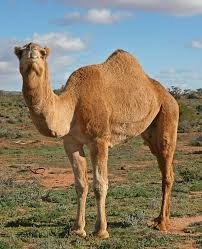Did you find an error or inaccuracy? Feel free to write us. Thank you!Tips to related online calculators
Do you have a linear equation or system of equations and looking for its solution? Or do you have a quadratic equation?
Do you want to convert velocity (speed) units?
Do you want to convert time units like minutes to seconds?

## Related math problems and questions:

• Cars 6At 9:00 am two cars started from the same town and traveled at a rate of 35 miles per hour and the other car traveled at a rate of 40 miles per hour. After how many hours will the cars be 30 miles apart?
• Two cars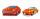From the town A to town B started two cars. The first at 7:00 at average speed 60 km per hour, the second at 10:00 at average speed 100 km per hour. The first car will not stay in B, and on the way back meet the second car at half way from A to B. At what
• Cars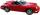Cars started at 7:00 AM. From point A car run at 40 km per hour, from B car run 70 km per hour. Between A and B is a 250 km long road. When and where will meet (measured from point A)?
• Two machinesThe first machine can produce parts in 24 hours, the second in 16 hours. The first started working at 6:00 pm. Second at 10:00 o'clock. At what hour were all parts made?
• StormSo far, a storm has traveled 35 miles in 1/2 hour in direction straight to observer. If it is currently 5:00 p. M. And the storm is 105 miles away from you, at what time will the storm reach you? Explain how you solved the problem.
• Cindy runSuppose it takes 1/3 of an hour for Cindy to run 3/5 of a mile. How many miles does she run in 1 hour?
• Runners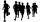If John has a running speed of 3.5miles per hour and Lucy has a speed of 5 miles per hour. If John starts running at 10:00 am, and Lucy starts running at 10:30 am, at what time will they meet? (as soon as possible)
• Train from BrnoFirst train started from Brno at 8 am at 60 km per hour. Second train started from Opava at 7 o'clock at the speed of 40 kilometers per hour. In how many hours and how far from cities trains meet when the distance of cities is 200 kilometers.
• A clockA clock was set right at 6:00 AM. If it gains 3 1/2 minutes per hour, what time will it show at 6:00 PM on the same day? Show your solution
• Brno-Tachov highwayThe distance Brno-Tachov is 360 km. At 8:00 a. M. , a car travels from Brno on the highway, traveling at an average speed of 90 km/h. An hour later, a car leaves Tachov towards Brno, whose average speed is 110 km/h. Calculate how far apart they will be at
• Train from PragueThe first train from Prague started at 8:00 hour at 40 kilometers per hour. Train from Ostrava started at 9:20 at 80 km per hour. How many hours and how far from cities with trains meet if the distance of cities is 400 km.
• Tourists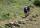From the cottage started first group of tourists at 7:00 AM at speed 4 km/h. The second started after them 21 minutes later at speed 6 km/h. How long and how many kilometers from the cottage will catch the first group?
• Walking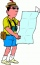Lucie can walk about 3 4/5 miles each hour. How far can she walk in 2 hours 45 minutes?
• The convoy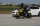During a military exercise, the convoy left the camp at 5 hours at a speed of 40 km/h. Half an hour later, a motorcycle clutch was sent at a speed of 70 km/h. How long before the motorcycle clutch reaches the column?
• RunningJoanne ran 8 1/2 Kyleigh 1 2/3 and John 6 3/4 miles in one day. How many miles did the girls run in 3 days?
• Two airportsTwo airports are 2690 km away. From the first airport, the airplane flies at a speed of 600 km/h, from a second airplane at a speed of 780 km/h. When will they meet if they flew at 10:00? How far from the first airport?
• Motion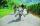Cyclist started at 9:00 from point S to point T. After 10 minutes, followed him at the same speed the second cyclist. Walker, which went from T to S, started at 9:35. After 71 minutes he met the first cyclist and after next 8 minutes the second cyclist. D